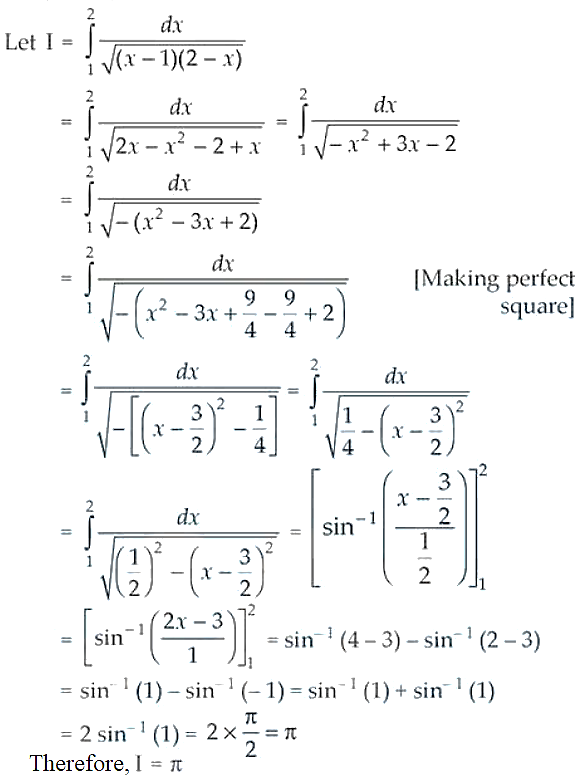# NCERT Exemplar Solutions for Class 12 Maths Chapter 7 Integrals

The NCERT Exemplar Solutions for Class 12 Maths Chapter 7 Integrals are available for students to get in-depth knowledge of the important concepts covered in this chapter. To help students solve the exemplar problems, the NCERT Exemplar Solutions have been created by subject experts at BYJU’S. These solutions are according to the latest CBSE syllabus and guidelines in order to help them score high marks in the annual examinations.

Chapter 7 of NCERT Exemplar Solutions for Class 12 Maths is Integrals. This chapter contains problems based on topics like integration, geometrical representation, properties of integrals, the comparison between differentiation and integration, different methods of integration, definite integral as the limit of a sum, properties of definite integrals, etc. To attain a good grip on solving problems of this chapter, students can download the PDF of NCERT Exemplar Solutions for Class 12 Maths Chapter 7 Integrals from the link given below and practise regularly.

## Download the PDF of NCERT Exemplar Solutions for Class 12 Maths Chapter 7 Integrals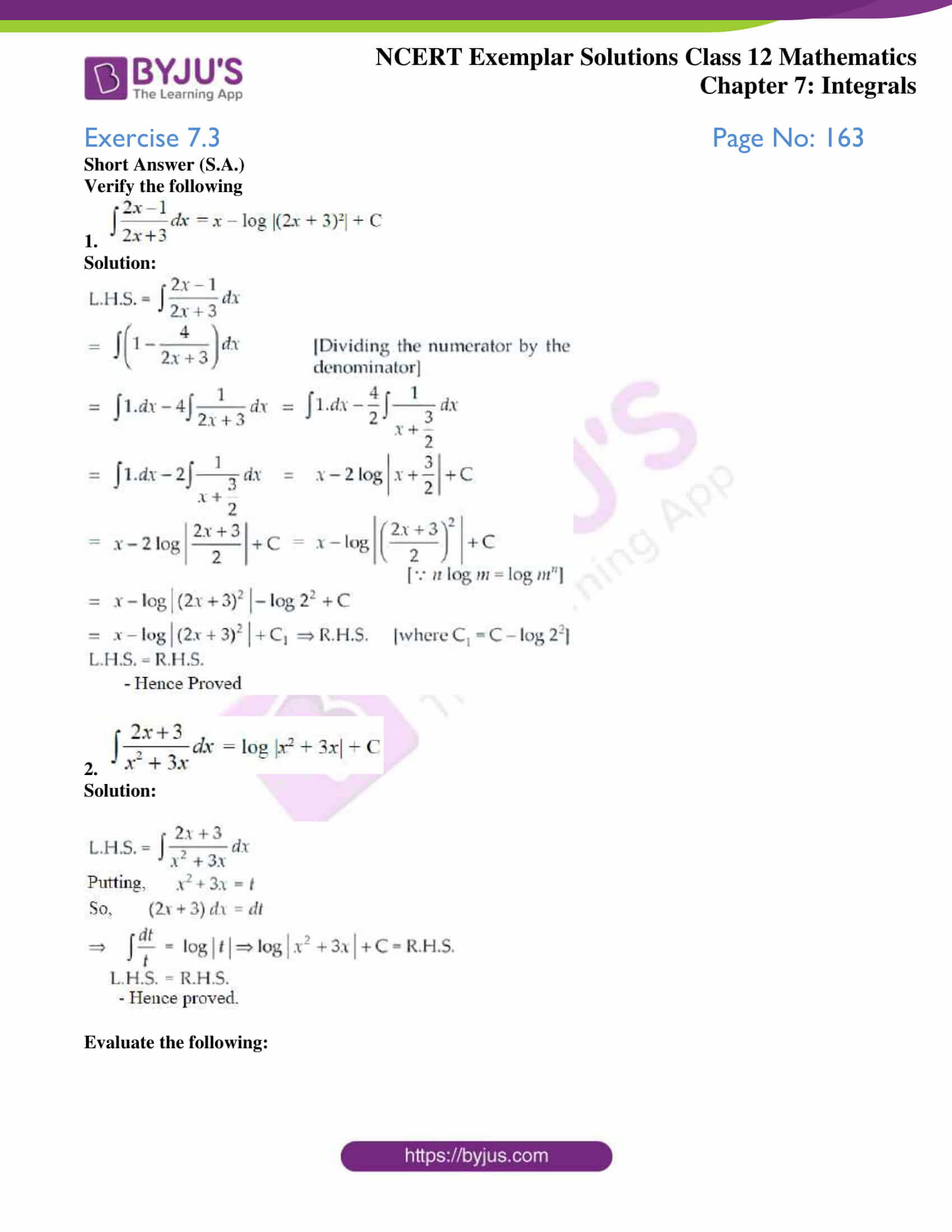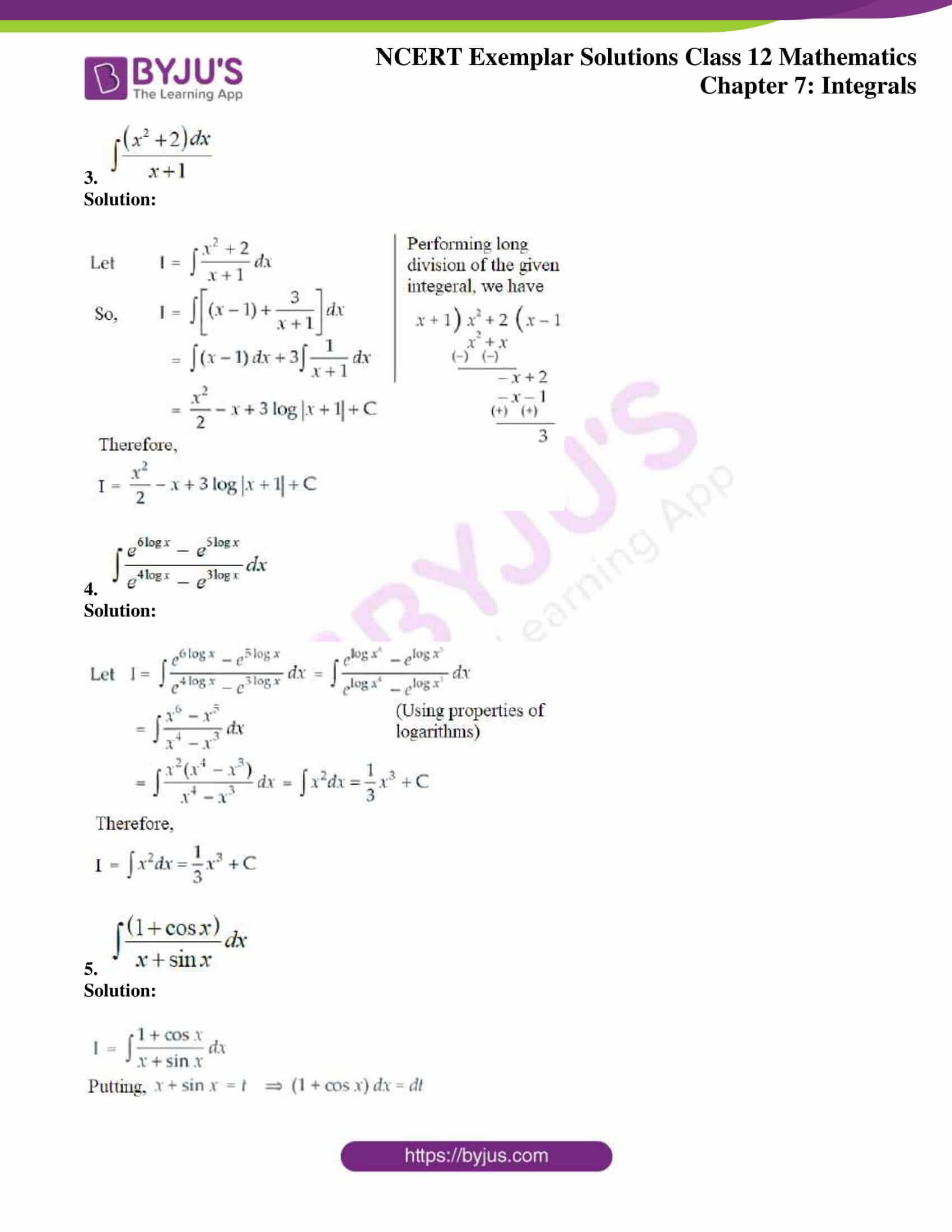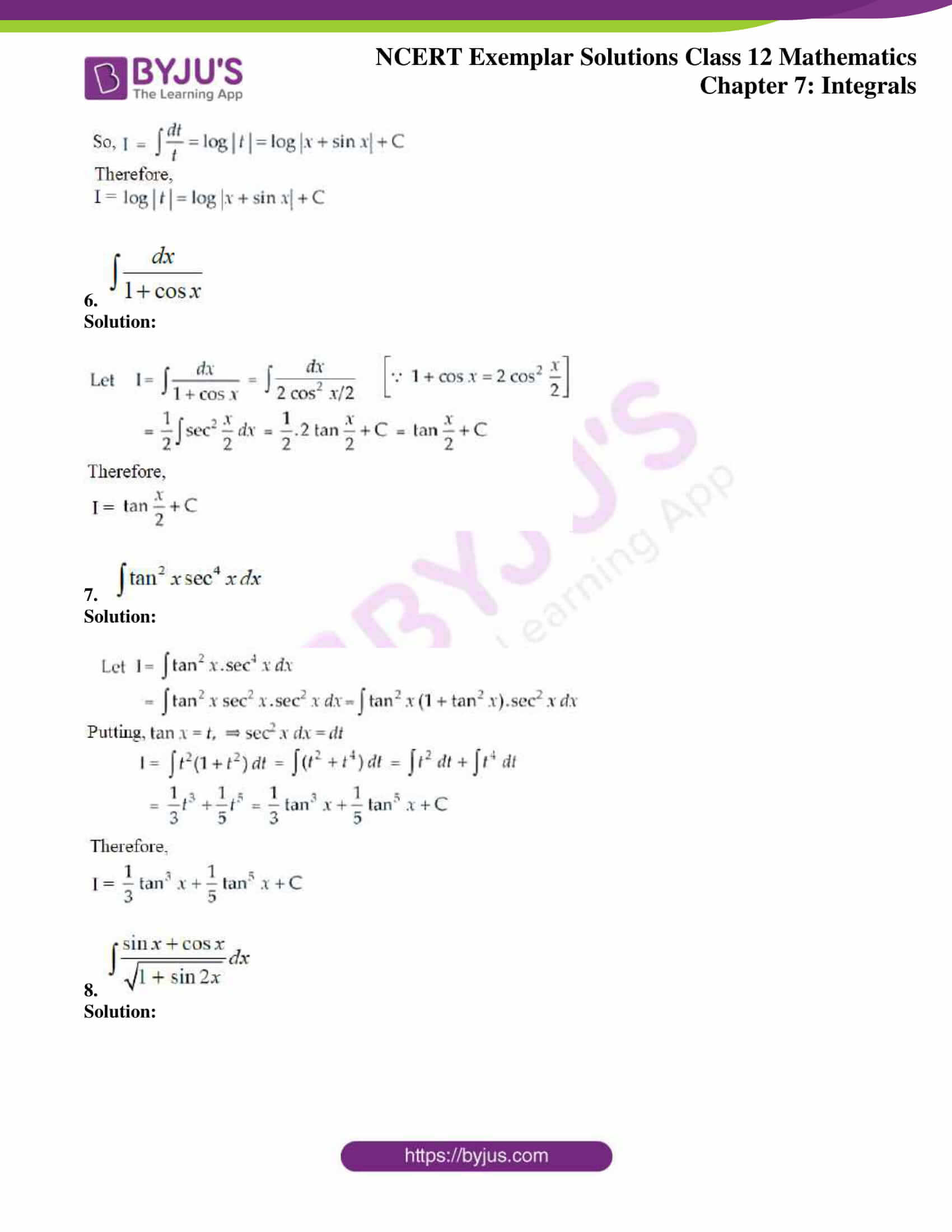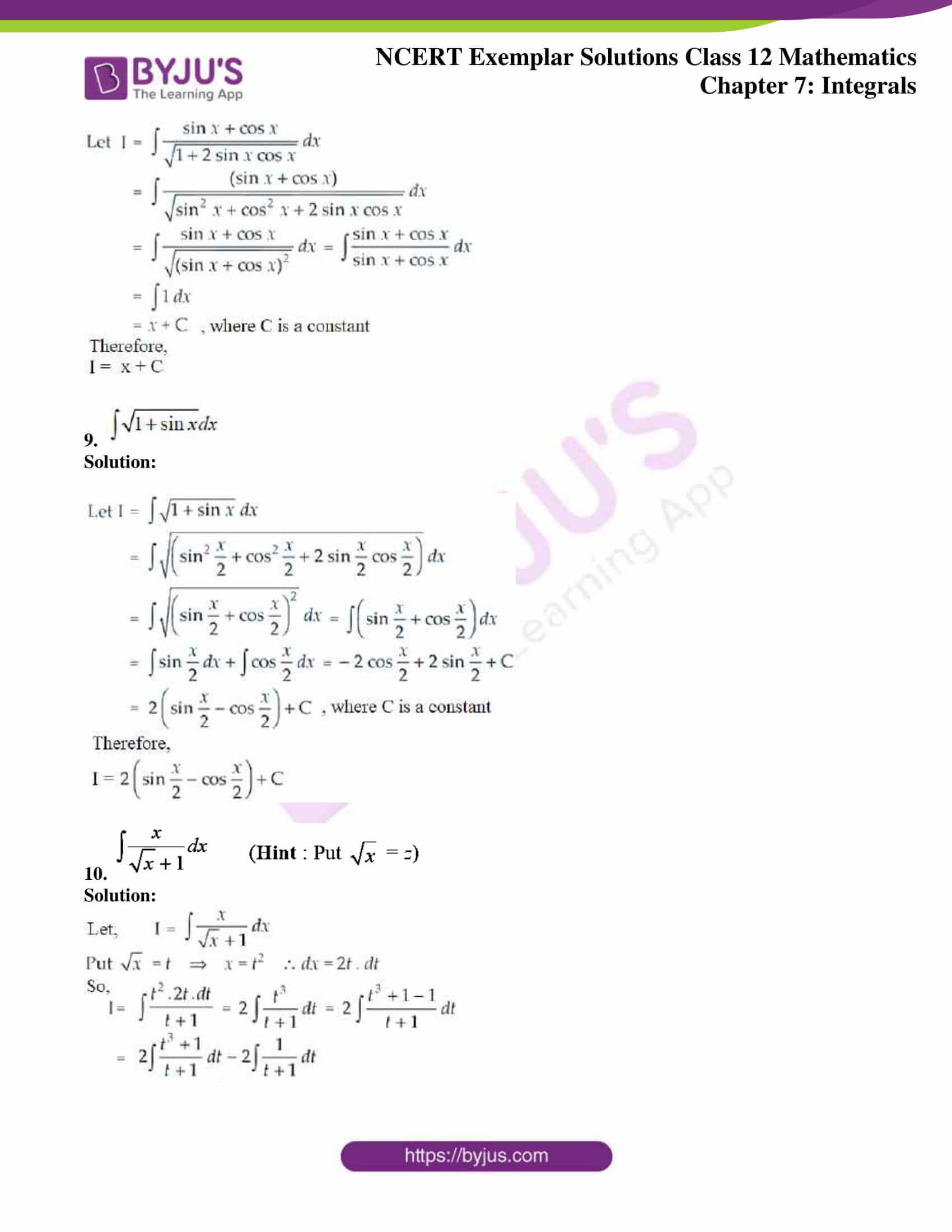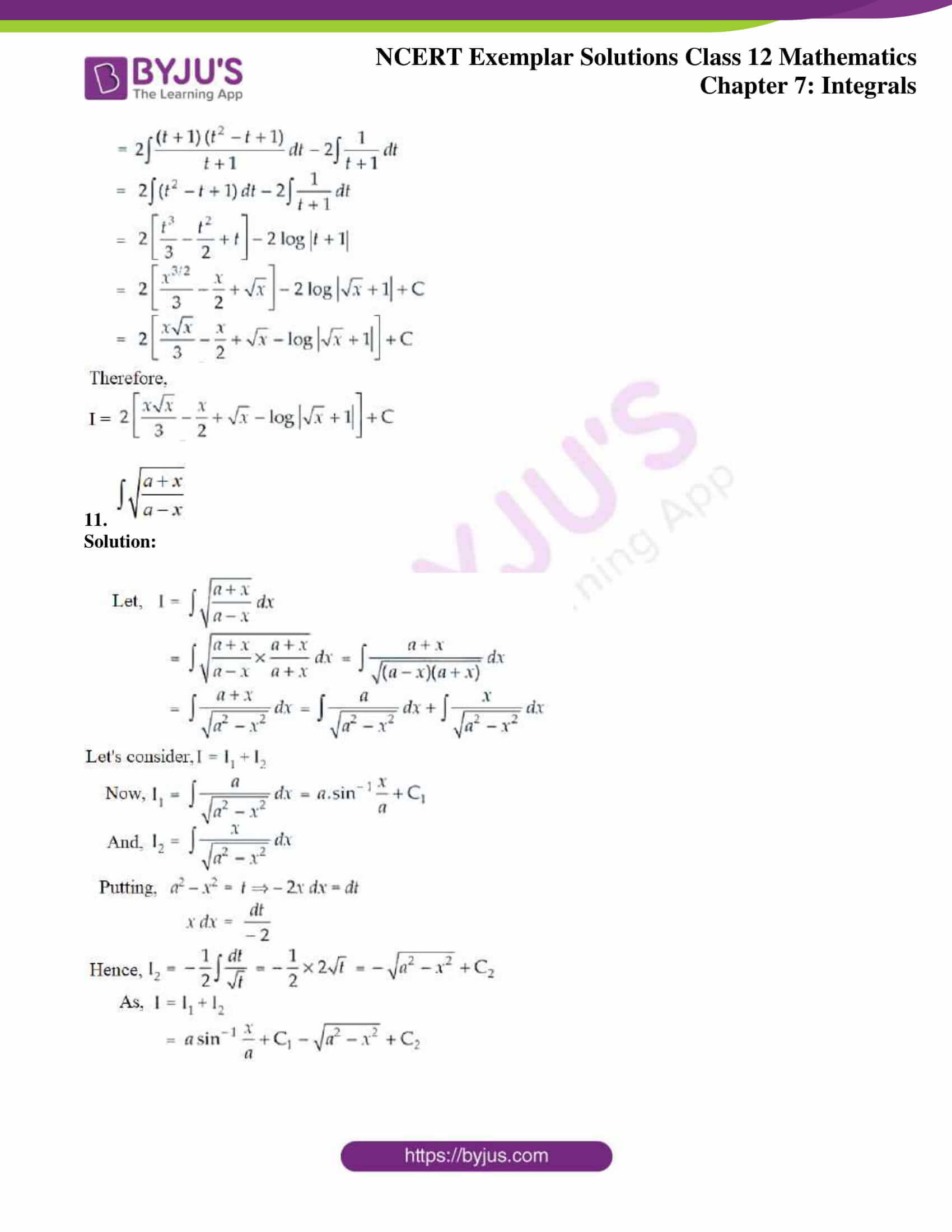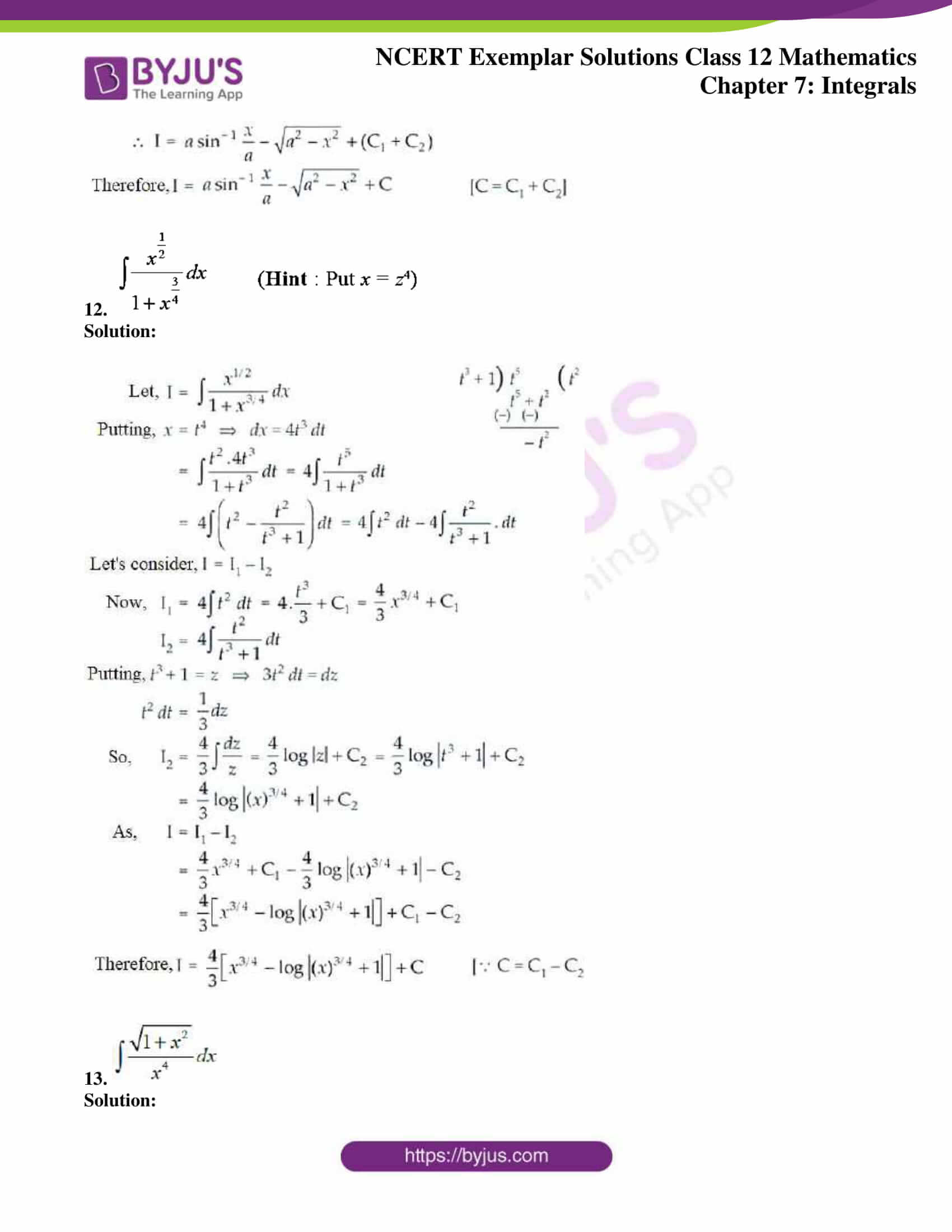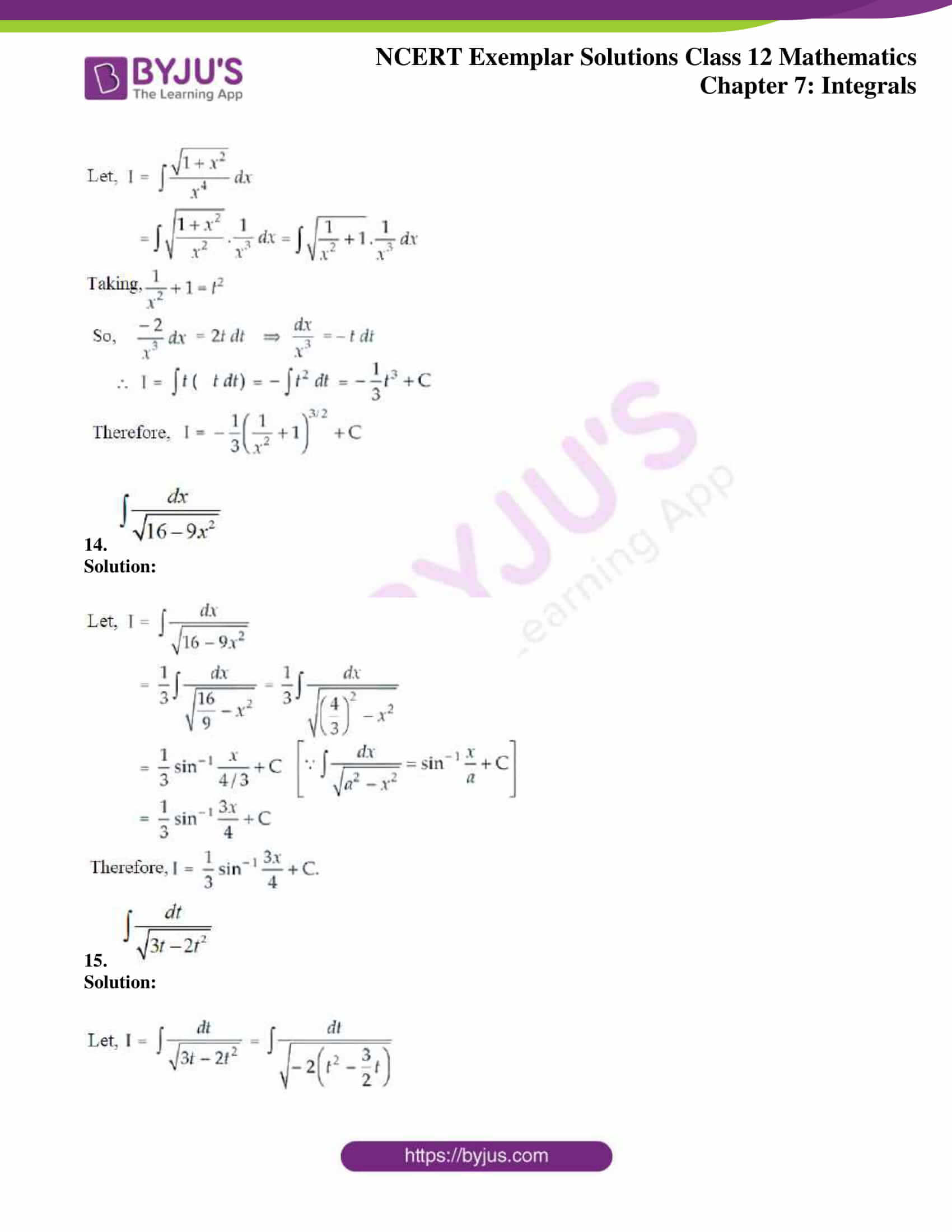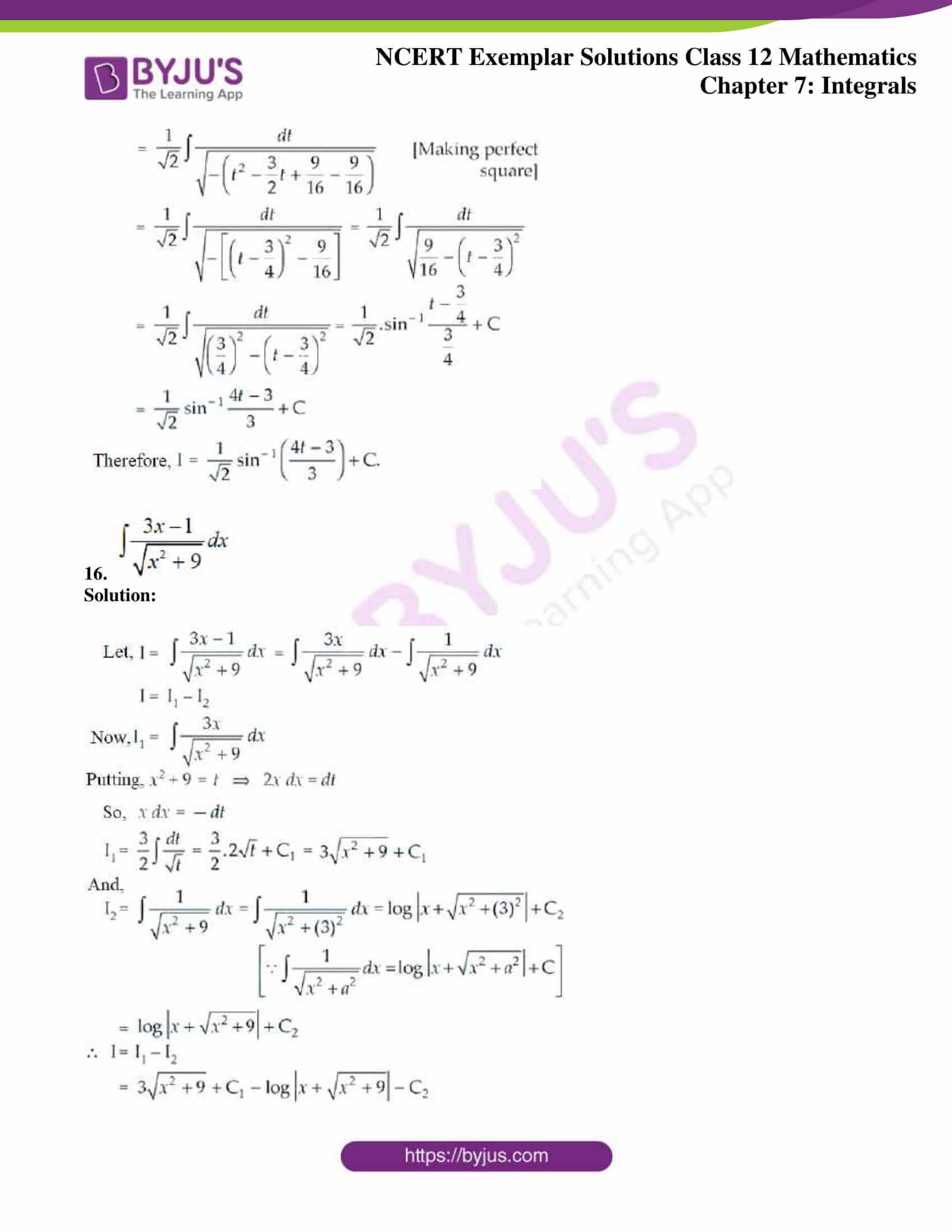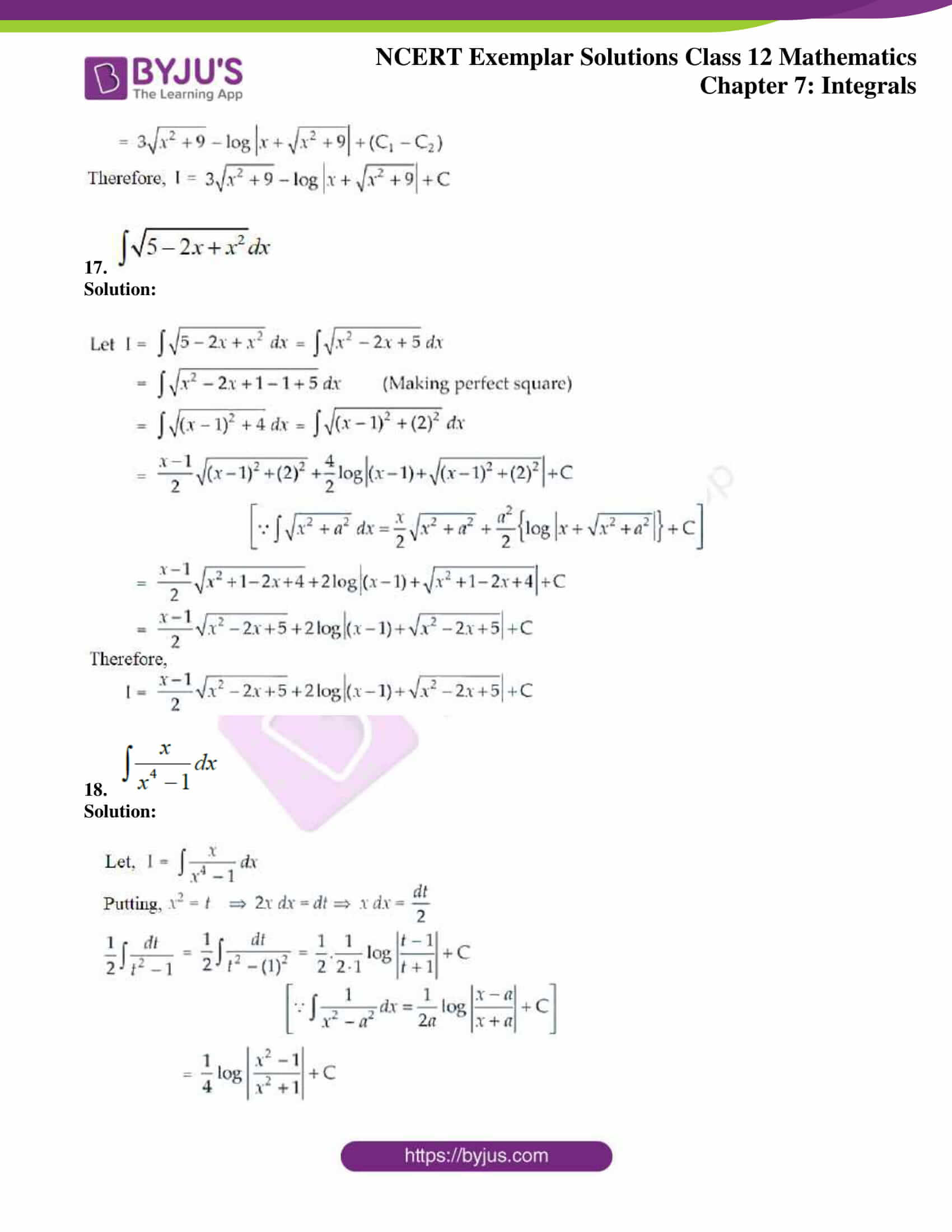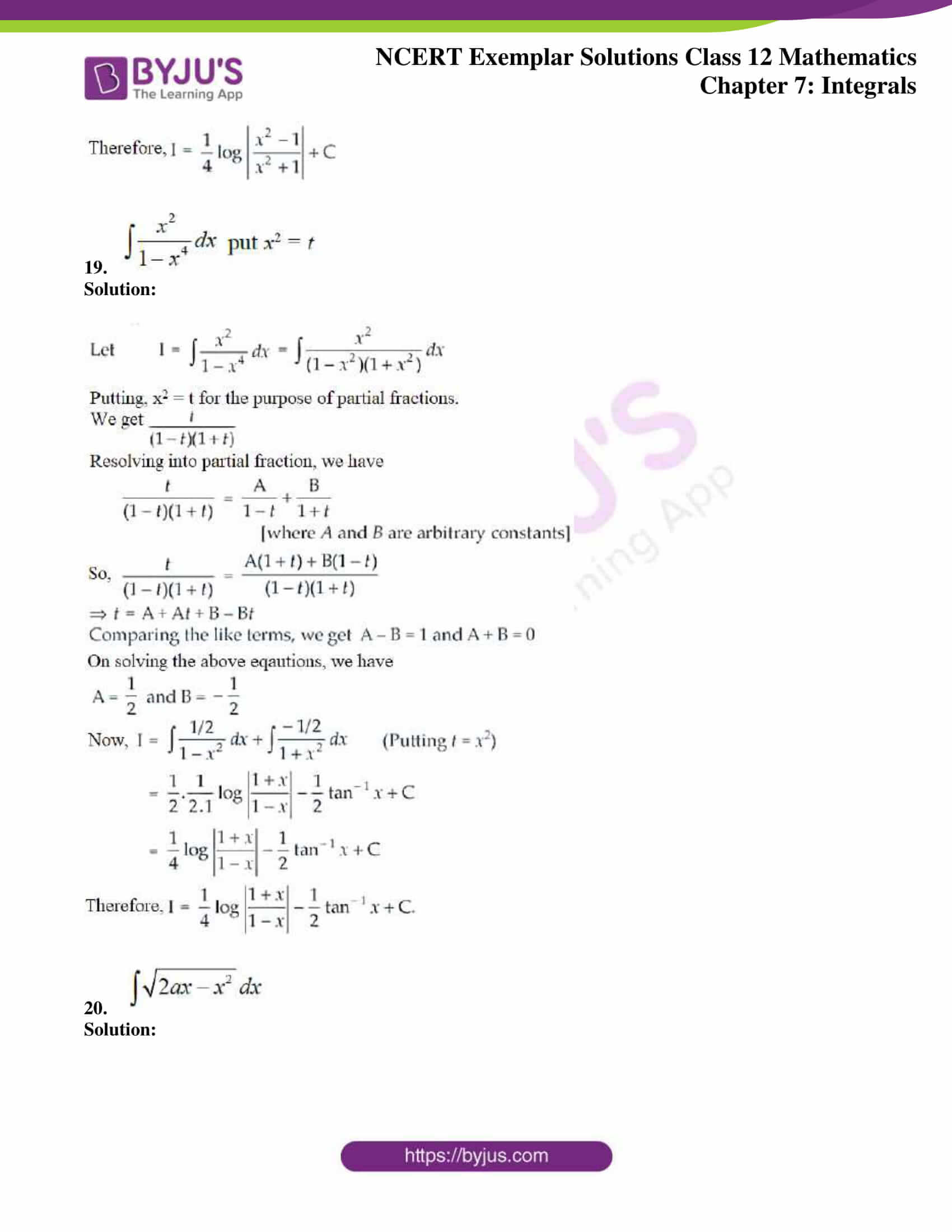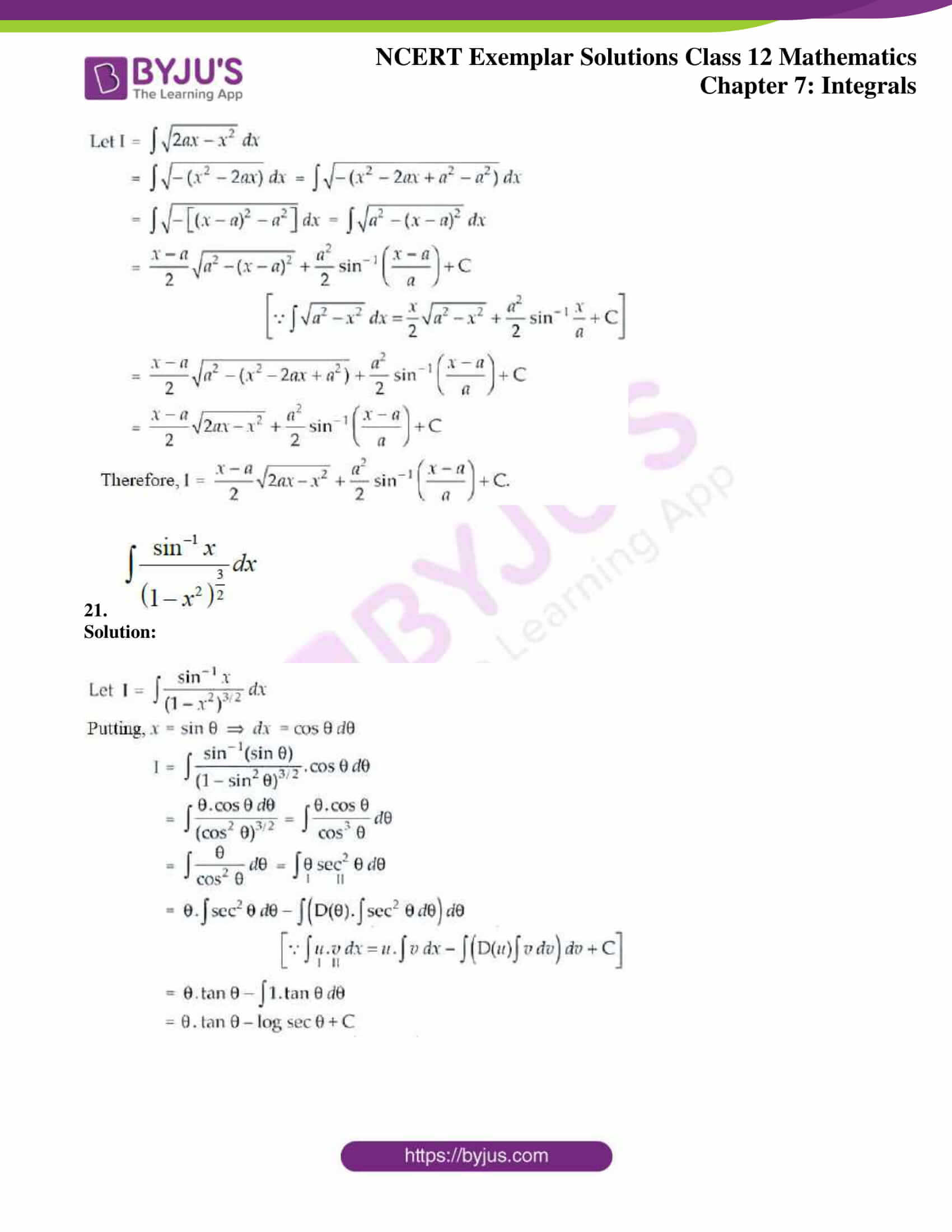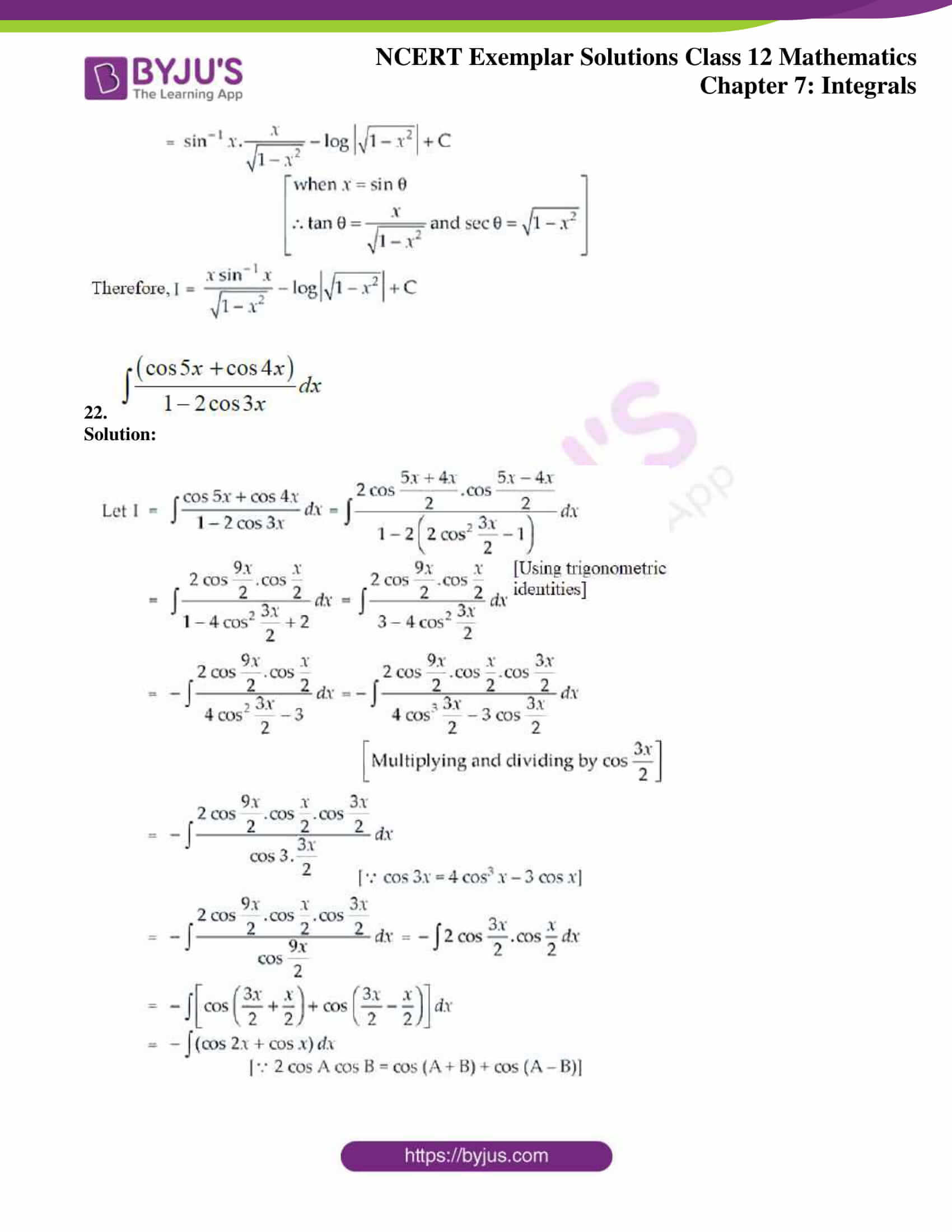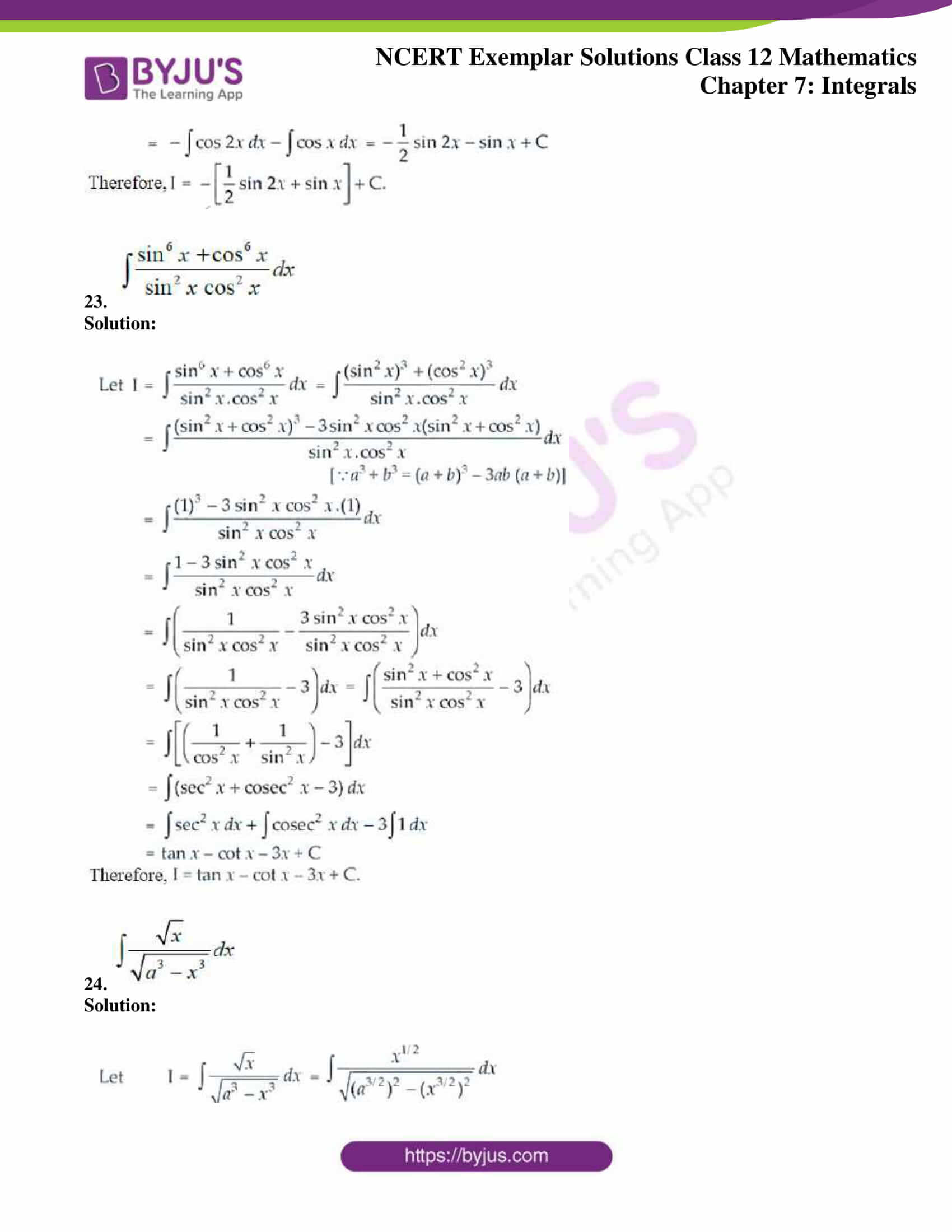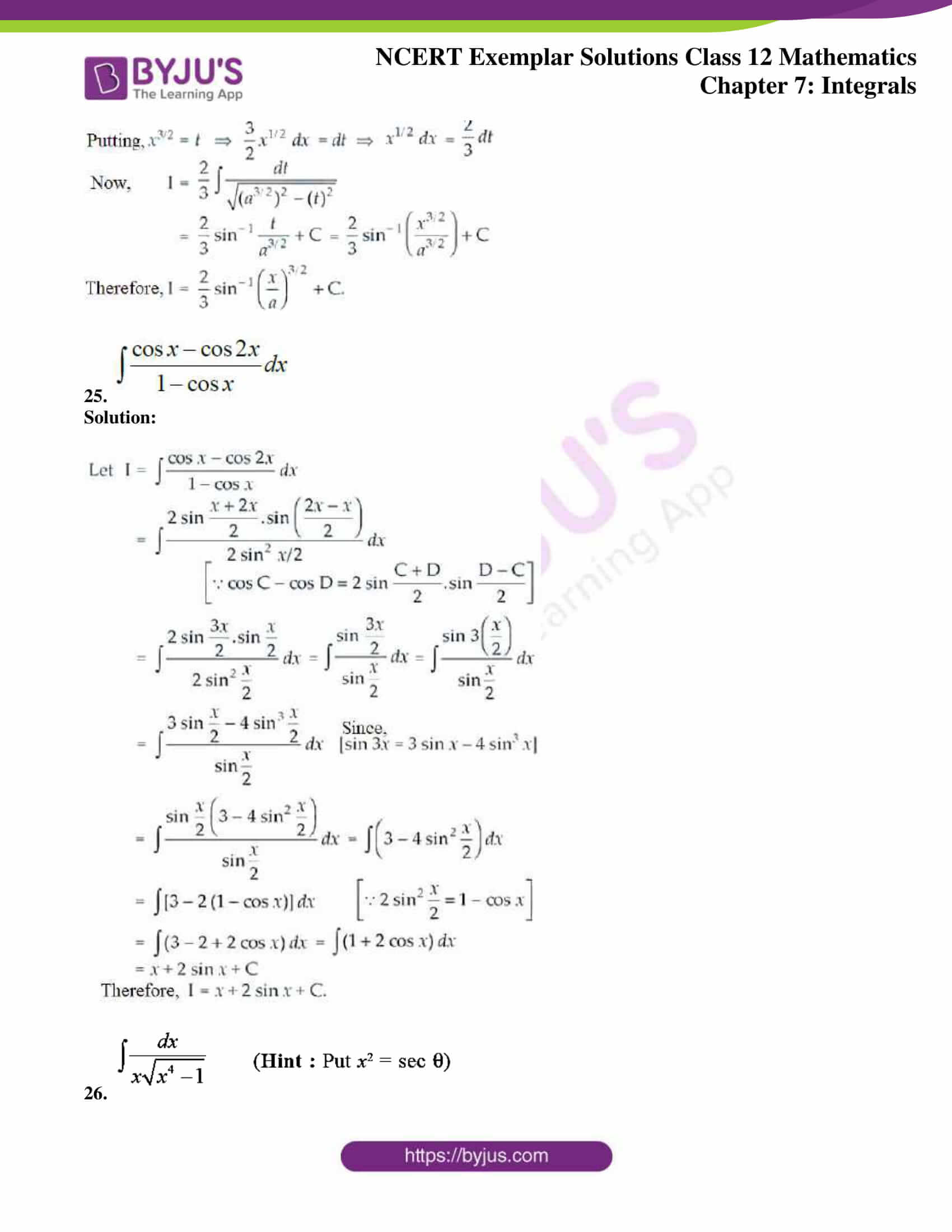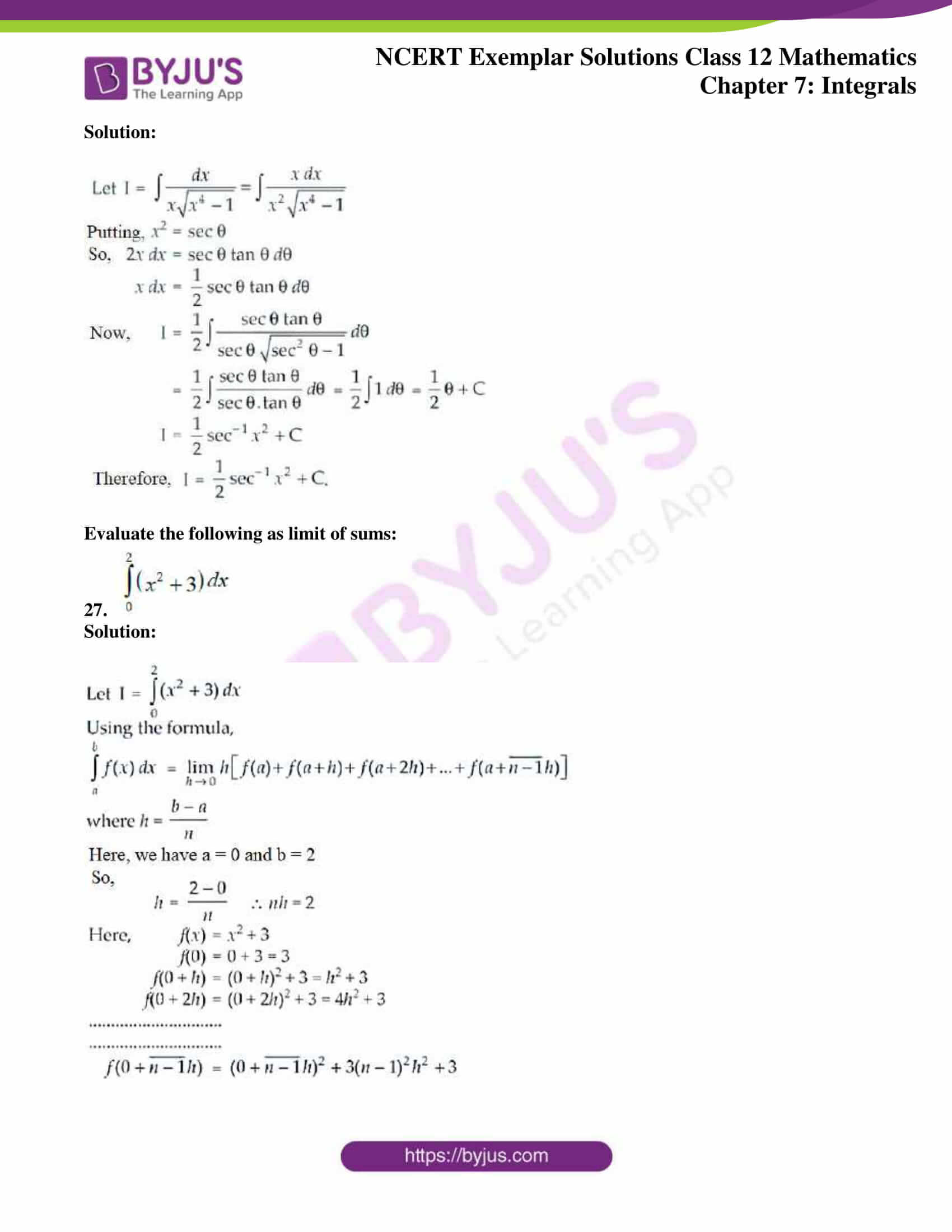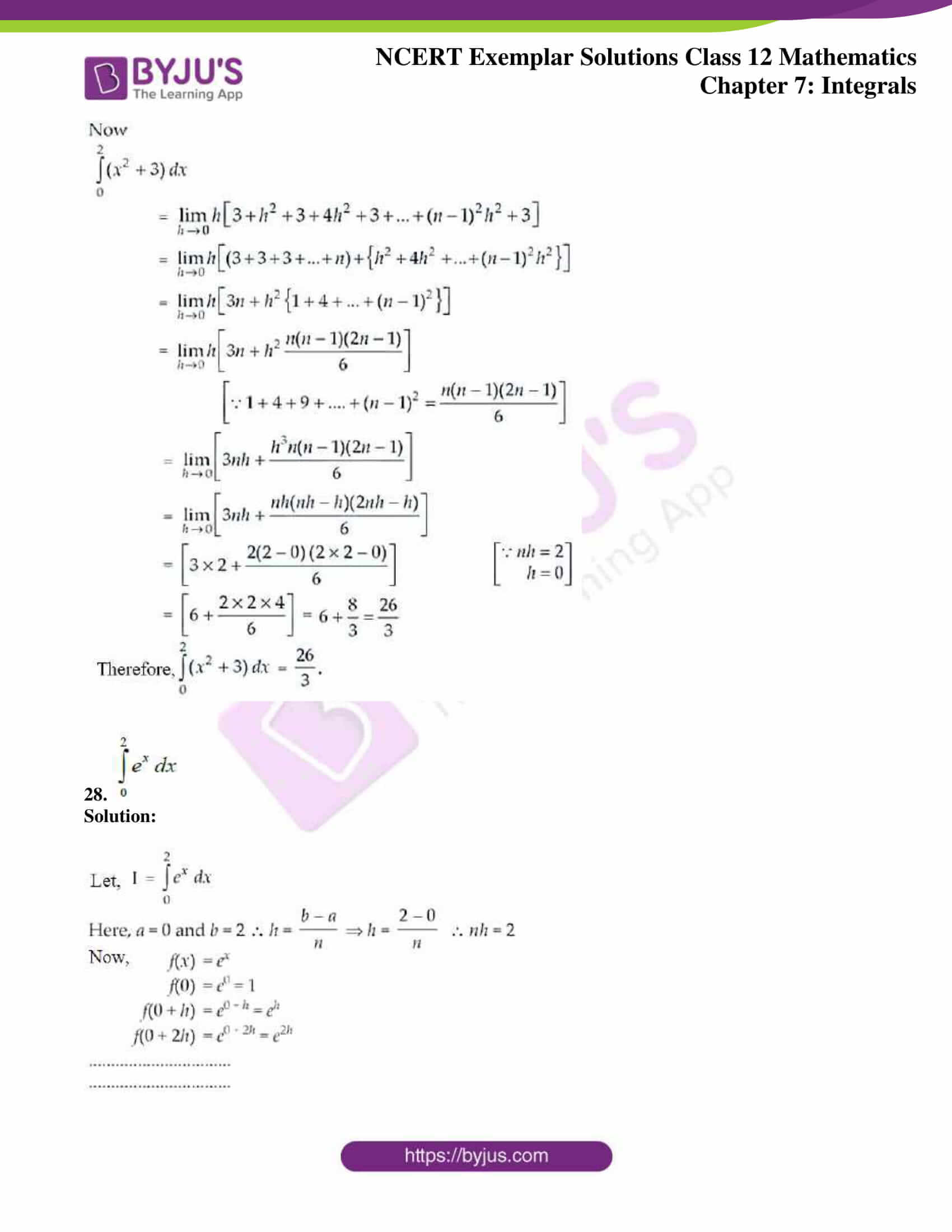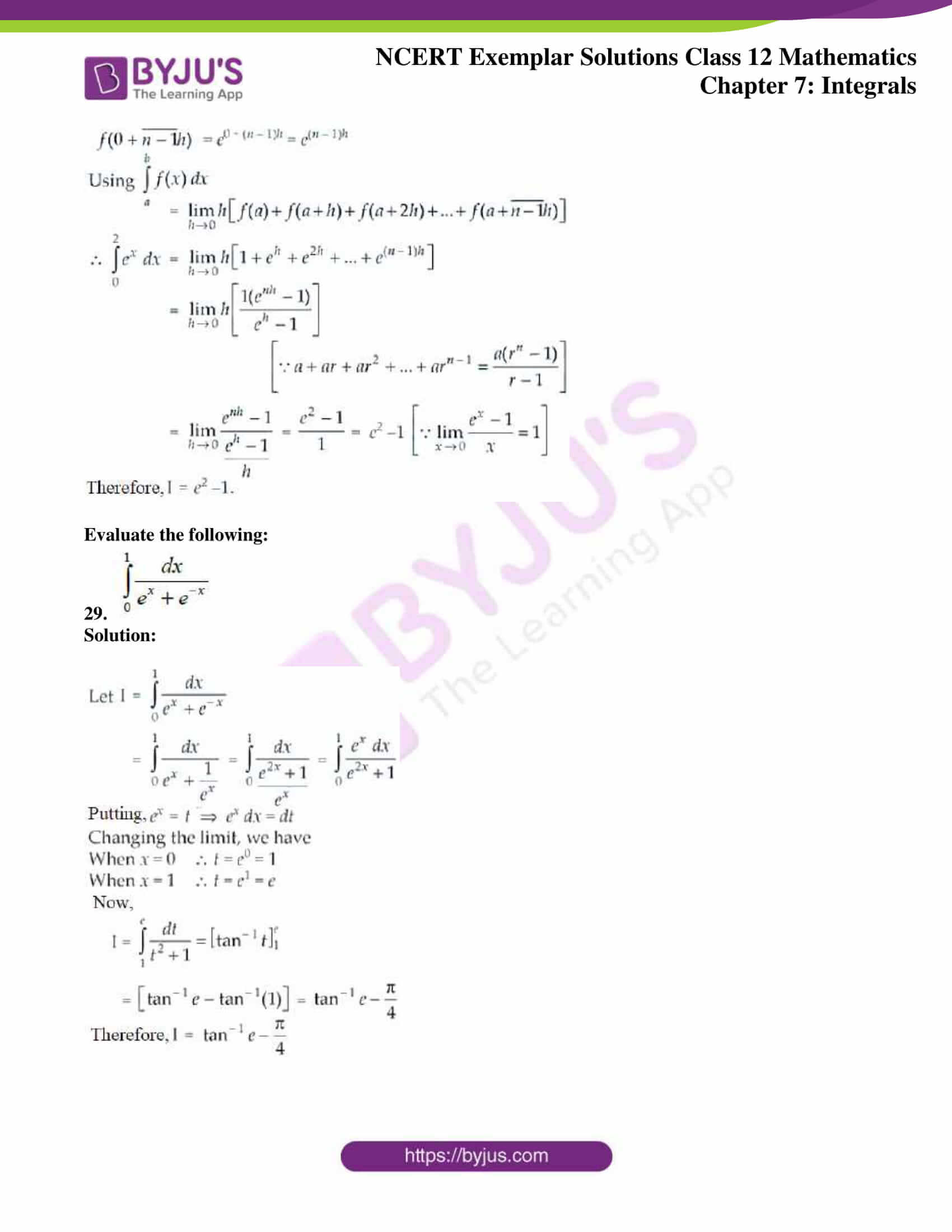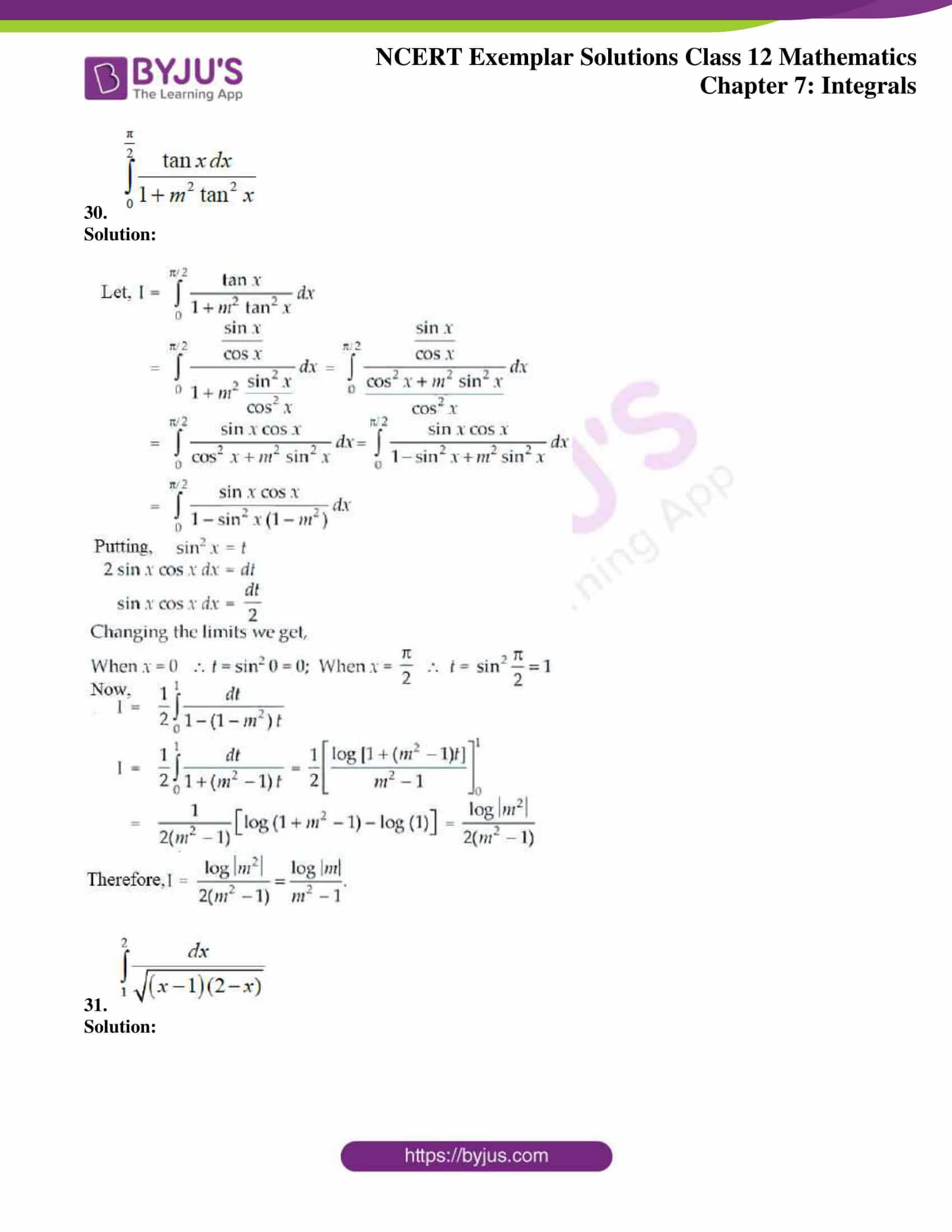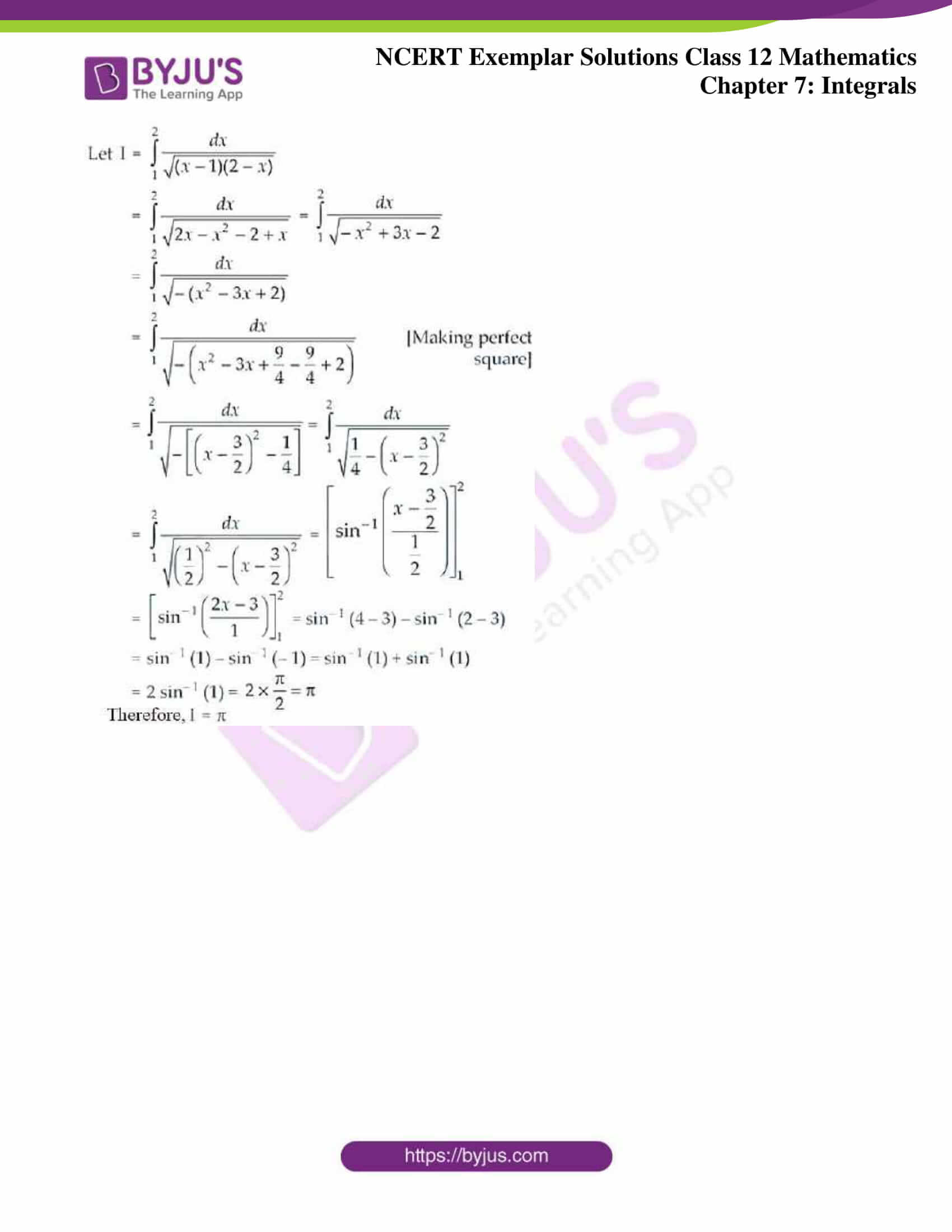### Access Answers to NCERT Exemplar Solutions for Class 12 Maths Chapter 7 Integrals

Exercise 7.3 Page No: 163

Verify the following

1.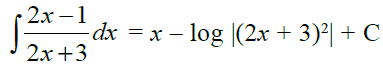Solution: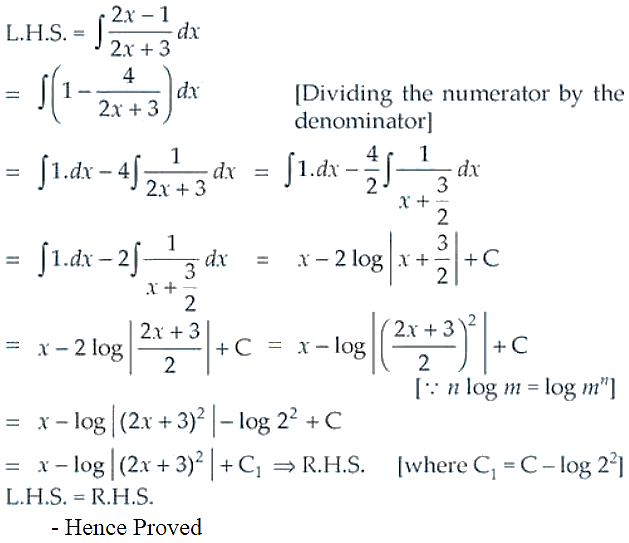2.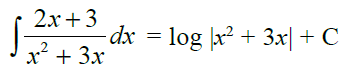Solution: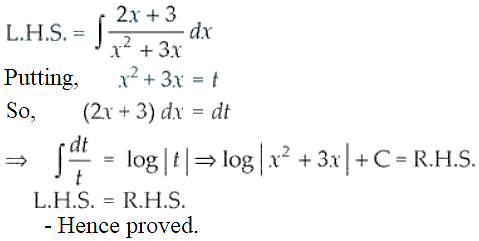Evaluate the following:

3.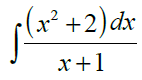Solution: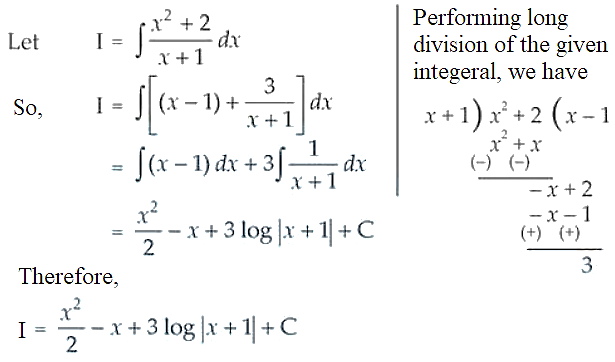4.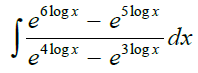Solution: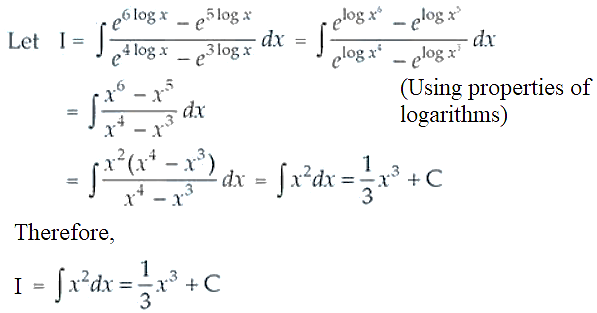5.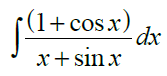Solution: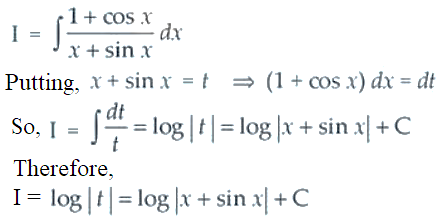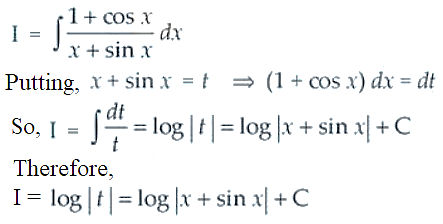6.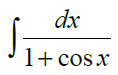Solution: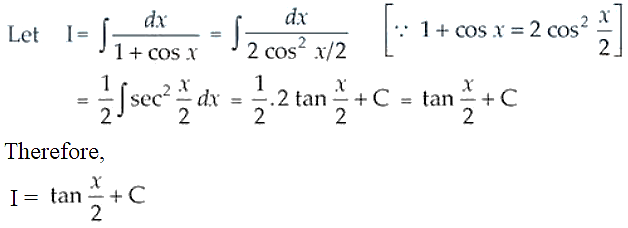7.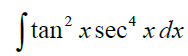Solution: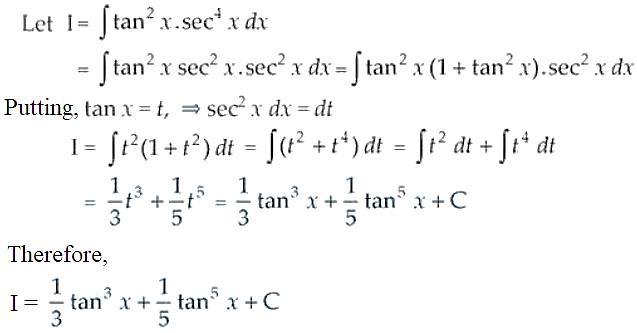8.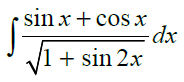Solution: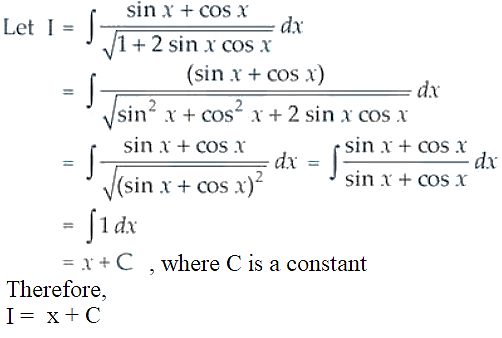9.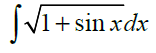Solution: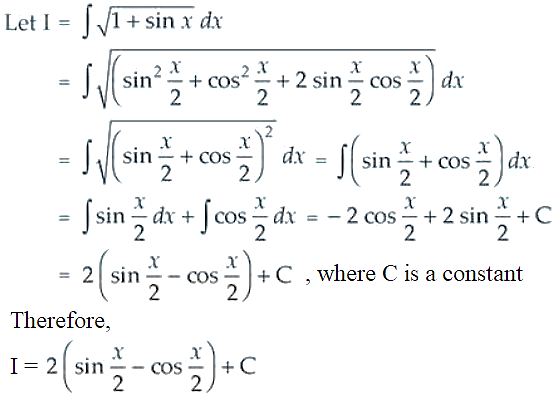10.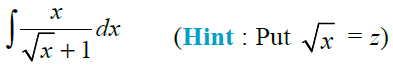Solution: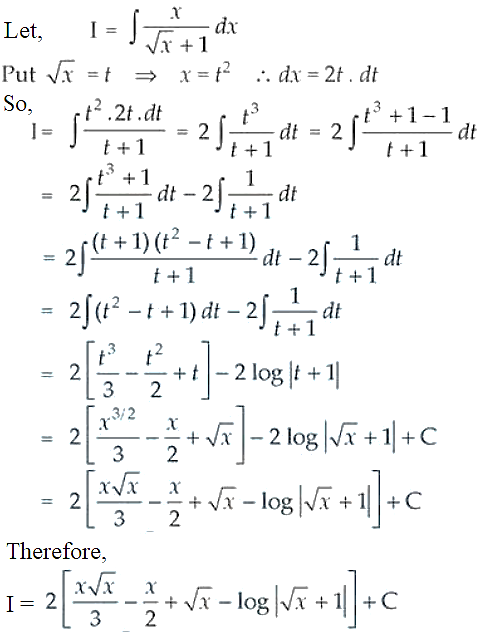11.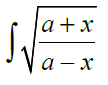Solution: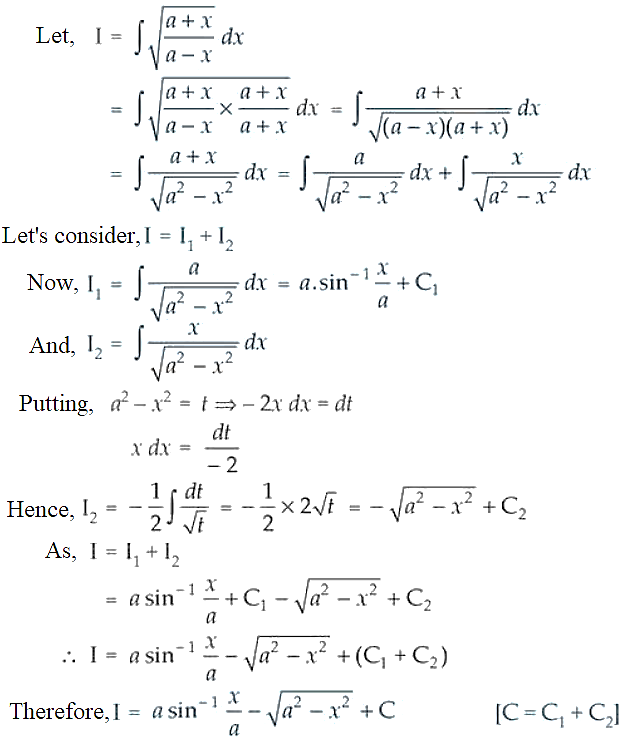12.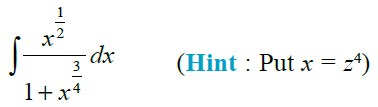Solution: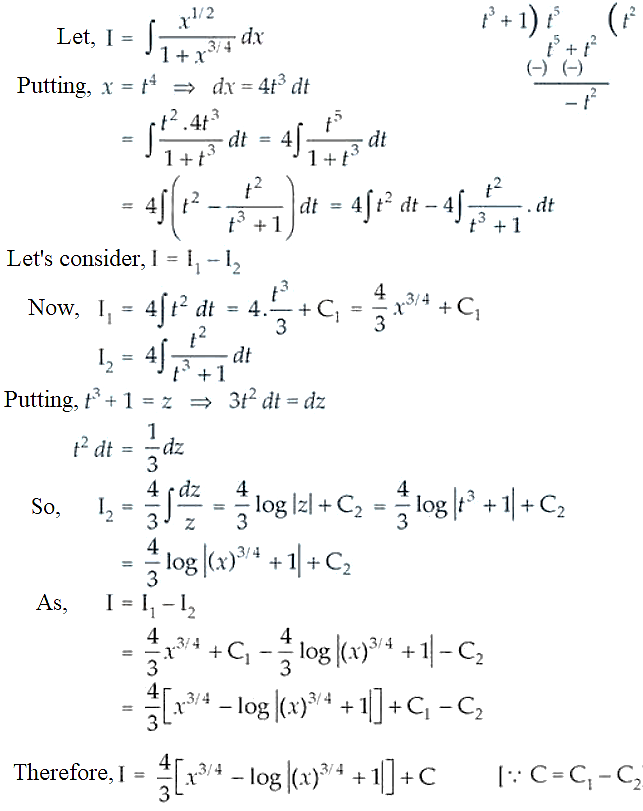13.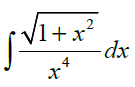Solution: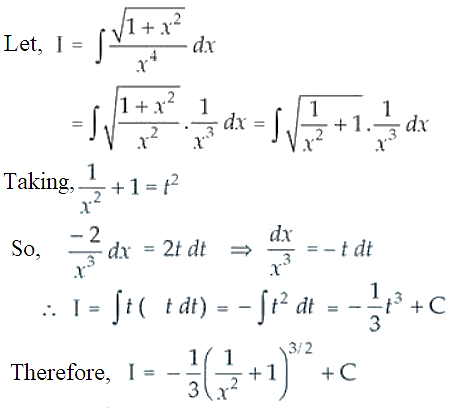14.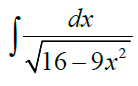Solution: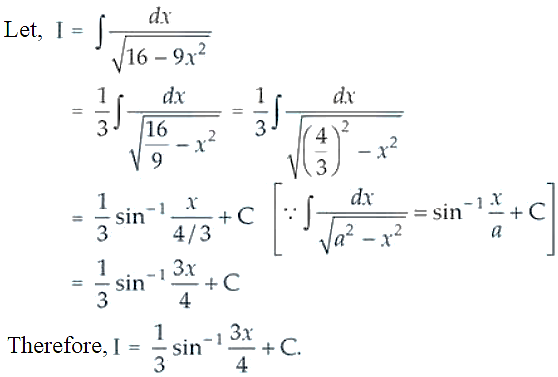15.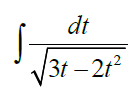Solution: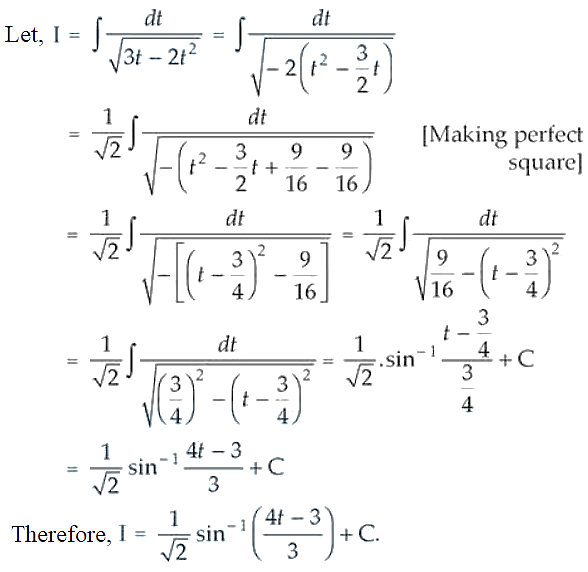16.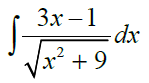Solution: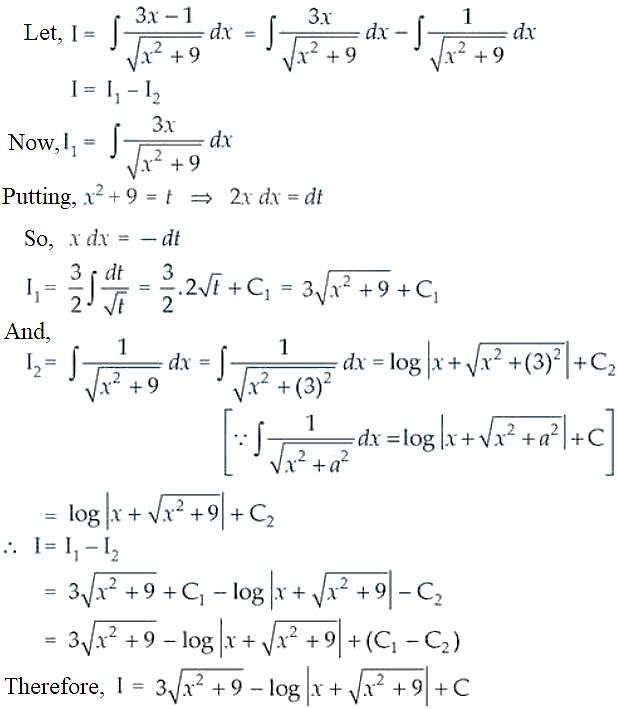17.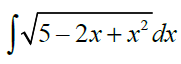Solution: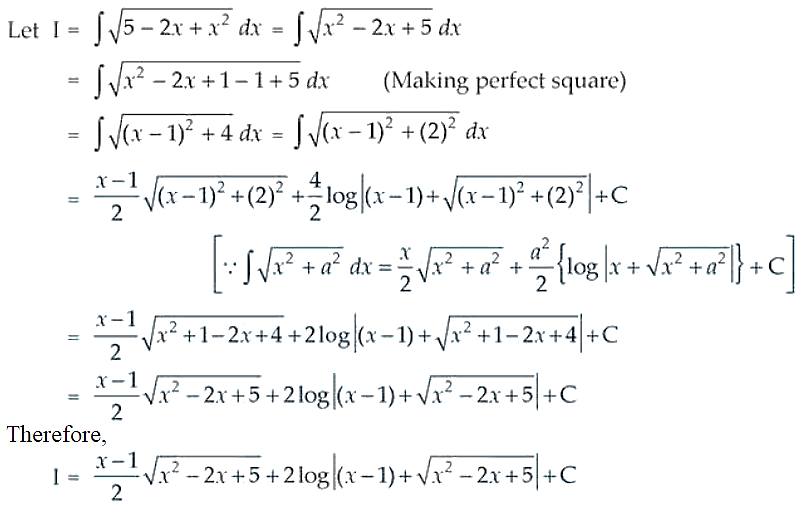18.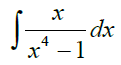Solution: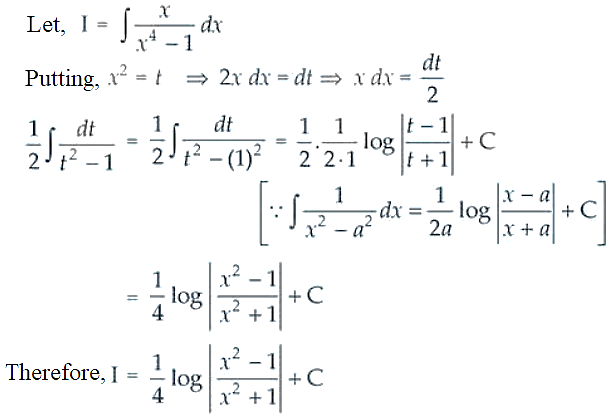19.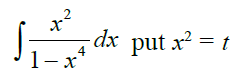Solution: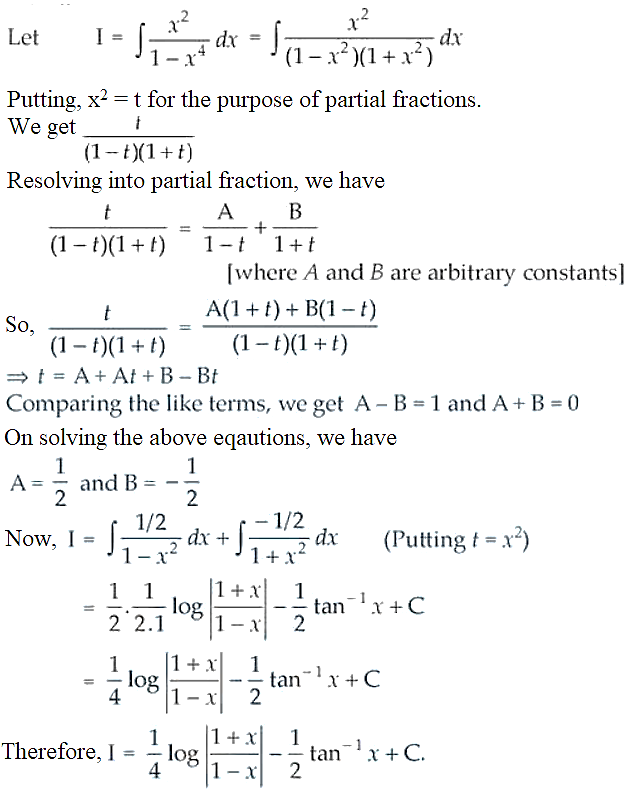20.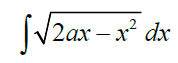Solution: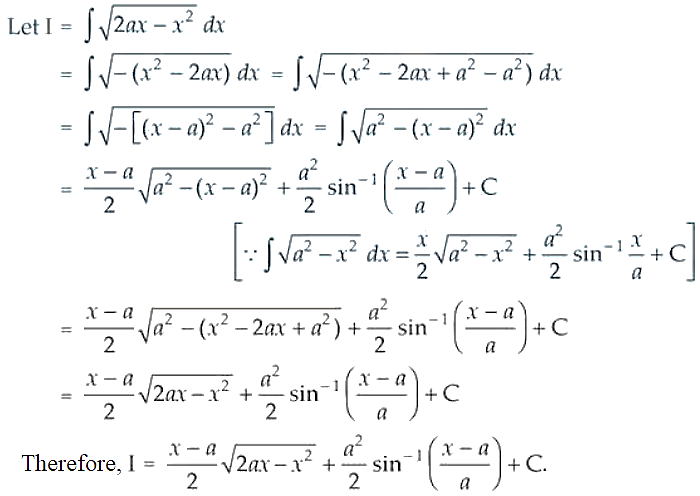21.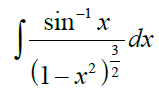Solution: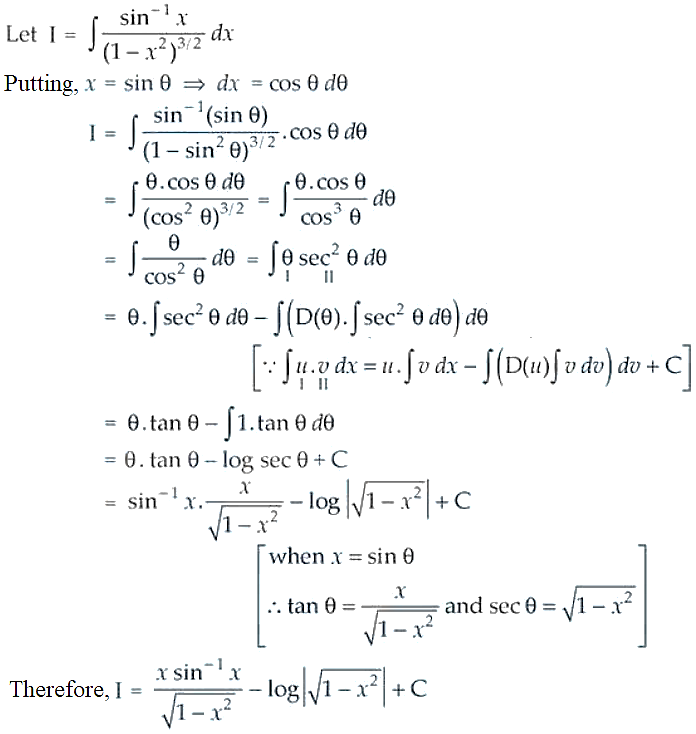22.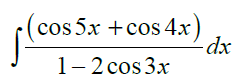Solution: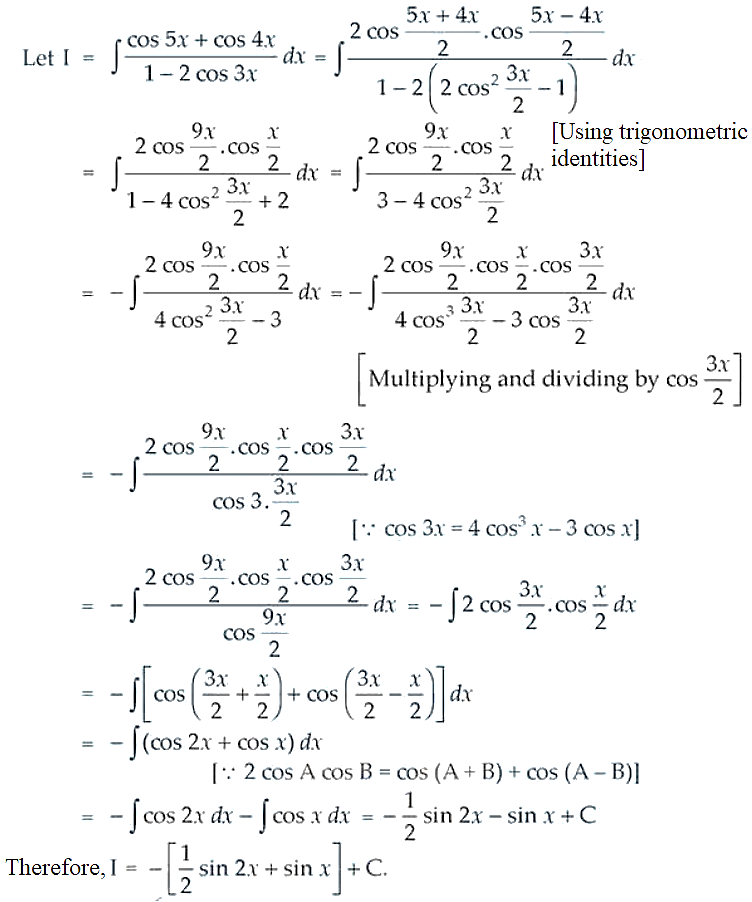23.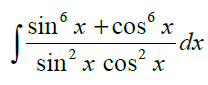Solution: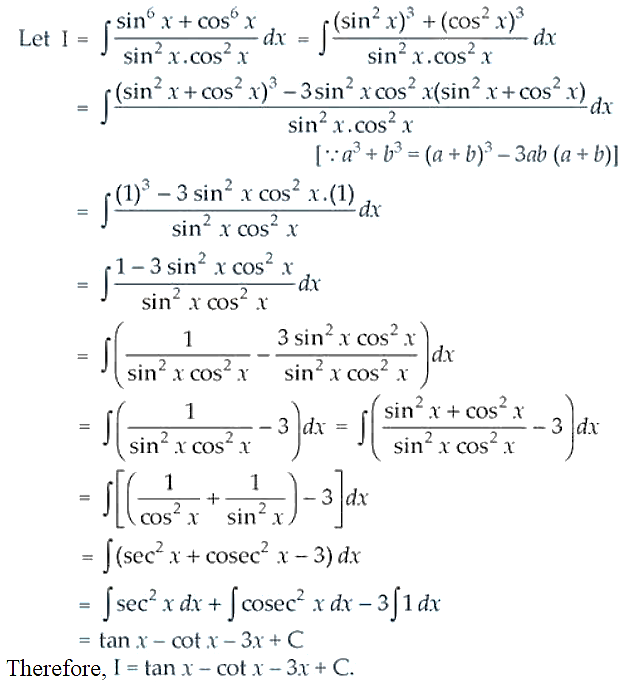24.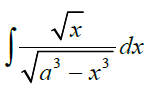Solution: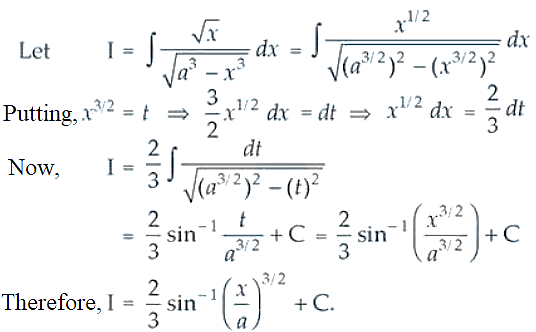25.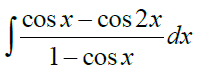Solution:26.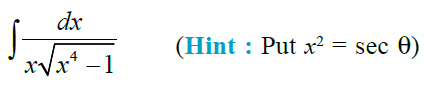Solution: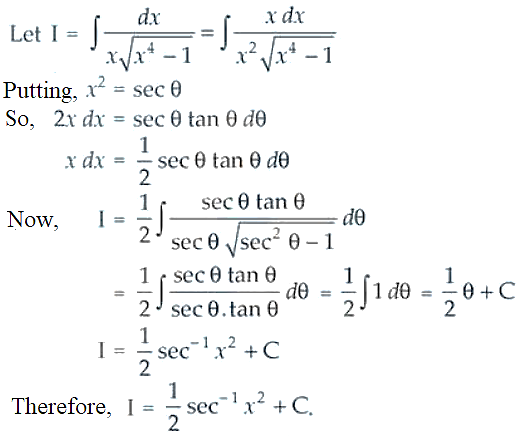Evaluate the following as limit of sums:

27.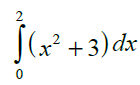Solution: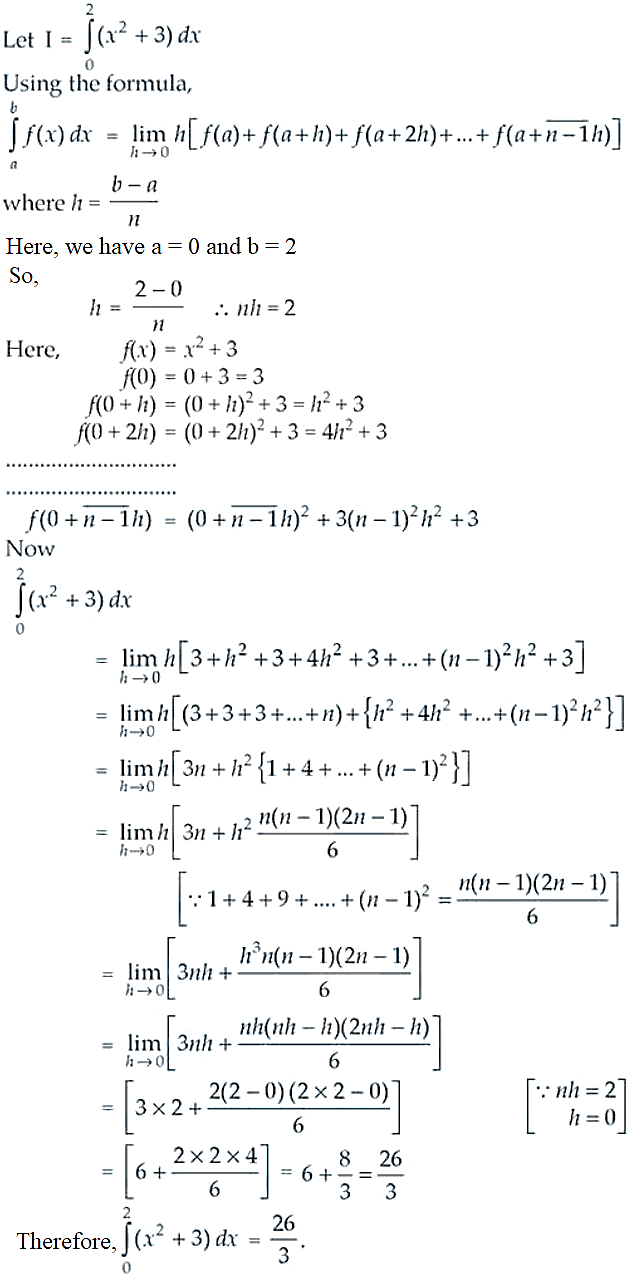28.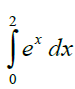Solution: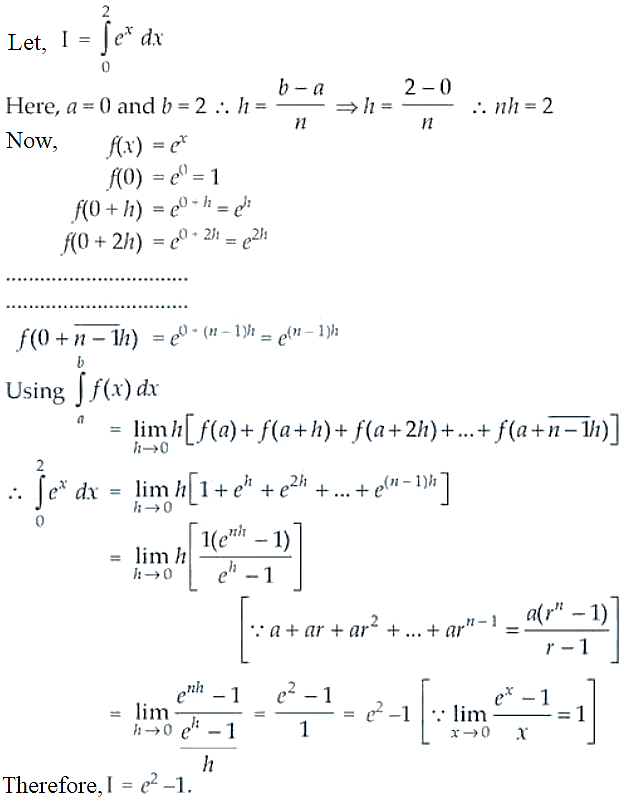Evaluate the following:

29.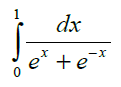Solution: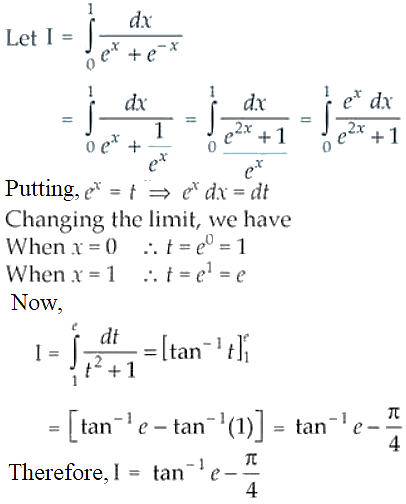30.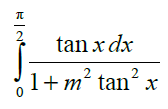Solution: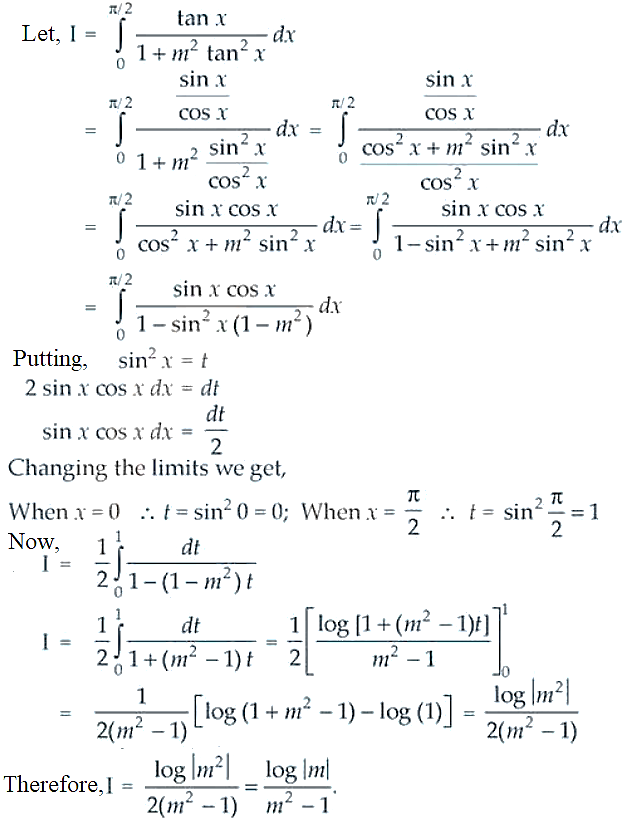31.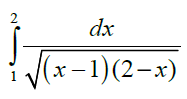Solution: